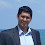## Friday, February 14, 2003

### Traveling Salesman on the Plane

Yesterday's post on NP-complete problems reminds me of one of the more interesting open questions, the complexity of the traveling salesman problem on the plane.

The traveling salesman problem consists of a collection of n cities, a symmetric distance function d(i,j) and a number k. The question is whether there is an ordering of the cities so that a tour through those cities and back to the start can be done in total distance at most k. If d takes on rational values, the problem is NP-complete in general and for many restrictions of the possible functions for d, such as requiring d to have the triangle inequality.

Consider the case where the cities are given as points (x,y) in the plane and the distance function is the regular Euclidean distance, i.e.,

d((r,s),(u,v))=((r-u)2+(s-v)2)1/2.
It is open whether this traveling salesman problem on the plane is NP-complete. In most cases, it is easy to show the problem is in NP and more difficult to show it NP-hard. For TSP on the plane, Garey, Graham and Johnson showed it NP-hard way back in 1976; it remains open whether the problem is in NP.

In NP one can guess the ordering of the cities, the problem is in checking whether the sum of distances is at most k. Since we can approximate the square root to a polynomial number of digits the issue is how many digits of accuracy we need. So it boils down to the following purely mathematical question: Given an expression of length m over integers with addition, subtraction, squares and square roots (but no recursive squares or square roots) what is the smallest positive number than can be expressed? If the answer is at least 2-mk for some k then TSP on the plane is in NP and NP-complete.

Let me also mention that one can well approximate traveling salesman on the plane.

1.2.